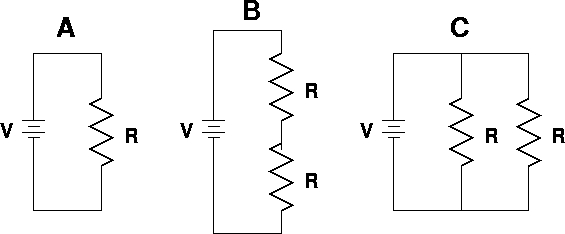# Problem: Consider the three circuits shown above. All the resistors and all the batteries are identical. (select true or false)a. The total power dissipated in circuit C is twice the total power dissipated in circuit B.b. The power dissipated in circuit A is twice the power dissipated in circuit B.c. The current through a resistor is the same in circuits A and C.d. The voltage across a single resistor in circuit C is twice the voltage across a single resistor in circuit B.e. The current through a resistor is the same in circuits A and B.

###### FREE Expert Solution

In this problem, we'll verify the statements by considering the following equations:

Power in circuits:

$\overline{){\mathbf{P}}{\mathbf{=}}{\mathbf{V}}{\mathbf{i}}{\mathbf{=}}{{\mathbf{i}}}^{{\mathbf{2}}}{\mathbf{R}}{\mathbf{=}}\frac{{\mathbf{V}}^{\mathbf{2}}}{\mathbf{R}}}$

Ohm's law:

$\overline{){\mathbit{i}}{\mathbf{=}}\frac{\mathbf{V}}{\mathbf{R}}}$

Equivalent resistance for 2 resistors in parallel:

$\overline{){{\mathbf{R}}}_{{\mathbf{eq}}}{\mathbf{=}}\frac{{\mathbf{R}}_{\mathbf{1}}{\mathbf{R}}_{\mathbf{2}}}{{\mathbf{R}}_{\mathbf{1}}\mathbf{+}{\mathbf{R}}_{\mathbf{2}}}}$

Equivalent resistance for resistors in series:

$\overline{){{\mathbf{R}}}_{{\mathbf{eq}}}{\mathbf{=}}{{\mathbf{R}}}_{{\mathbf{1}}}{\mathbf{+}}{{\mathbf{R}}}_{{\mathbf{2}}}{\mathbf{+}}{\mathbf{.}}{\mathbf{.}}{\mathbf{.}}{\mathbf{+}}{{\mathbf{R}}}_{{\mathbf{n}}}}$

94% (77 ratings)###### Problem DetailsConsider the three circuits shown above. All the resistors and all the batteries are identical.

(select true or false)

a. The total power dissipated in circuit C is twice the total power dissipated in circuit B.
b. The power dissipated in circuit A is twice the power dissipated in circuit B.
c. The current through a resistor is the same in circuits A and C.
d. The voltage across a single resistor in circuit C is twice the voltage across a single resistor in circuit B.
e. The current through a resistor is the same in circuits A and B.

Frequently Asked Questions

What scientific concept do you need to know in order to solve this problem?

Our tutors have indicated that to solve this problem you will need to apply the Power in Circuits concept. You can view video lessons to learn Power in Circuits. Or if you need more Power in Circuits practice, you can also practice Power in Circuits practice problems.